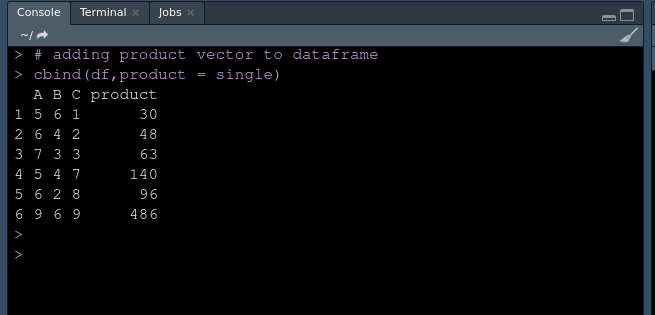Skip to content
Related Articles
Apply Function to each Row in R DataFrame
• Last Updated : 29 May, 2021

In this article, we will discuss how to apply a function to each row in a data frame in R Programming Language. Let’s take an example for better understanding.

Example:

Suppose we have a dummy dataset with A, B, C as column names and some numeric value as rows.

And we want to apply a function that will return the product of each row values, then the resultant data frame should look like this:

### Apply function to each row in R Data frame:

Approach: Using apply function

apply() is used to compute a function on a data frame or matrix. The purpose of using apply() function is to avoid the use of looping. apply() function returns output as a vector.

Syntax: apply(x, margin, func)

Parameters:
x: Array or matrix
margin: dimension on which operation is to be applied
func: operation to be applied

Stepwise implementation:

Step 1: Create a dummy dataset.

## R

 `# Apply function to each row in r Dataframe` `# Creating dataset``# creating firs column``x <- ``c``(5, 6, 7, 5, 6, 9)` `# creating second column``y <- ``c``(6, 4, 3, 4, 2, 6)` `# creating third column``z <- ``c``(1, 2, 3, 7, 8, 9)` `# creating dataframe``df <- ``data.frame``(A = x, B = y, C = z)` `display``(df)`

Output:

```  A B C
1 5 6 1
2 6 4 2
3 7 3 3
4 5 4 7
5 6 2 8
6 9 6 9```

Step 2: Create a custom function for calculating products.

## R

 `# creating function to computer product``product = ``function``(x, output){``  ` `  ``# accessing elements from first column``  ``A = x``  ` `  ``# accessing elements from second column``  ``B=x``  ` `  ``# accessing elements from third column``  ``C= x``  ` `  ``# return product``  ``return``(A*B*C)``}`

Note: Here we are just defining the function for computing product and not calling, so there will be no output until we call this function.

Step 3: Use apply the function to compute the product of each row.

Syntax: (data_frame, 1, function,…)

Now we are calling the newly created product function and returns the product using apply function.

## R

 `# apply(X,MARGIN,FUN,...)``apply``(df,1,product  )`

Output:

`  30  48  63 140  96 486`

Note: apply() return product as a vector list. So we have to add it to the data frame using cbind (column bind).

Step 4: Append product list to the data frame.

## R

 `# apply(X,MARGIN,FUN,...)``single <- ``apply``(df,1,product  )` `# adding product vector to dataframe``cbind``(df,product = single)`

Output:Using apply()

Below is the full implementation:

## Python3

 `# Apply function to each row in r Dataframe` `# Creating dataset``# creating firs column``x <``-` `c(``5``,``6``,``7``,``5``,``6``,``9``)` `# creating second column``y <``-` `c(``6``,``4``,``3``,``4``,``2``,``6``)` `# creating third column``z <``-` `c(``1``,``2``,``3``,``7``,``8``,``9``)` `# creating dataframe``df <``-` `data.frame(A``=``x,B``=``y,C``=``z)` `# creating function to computer product``product ``=` `function(x,output){``  ` `  ``# accessing elements from first column``  ``A ``=` `x[``1``]``  ` `  ``# accessing elements from second column``  ``B``=``x[``2``]``  ` `  ``# accessing elements from third column``  ``C``=` `x[``3``]``  ` `  ``# return product``  ``return``(A``*``B``*``C)``}` `# apply(X,MARGIN,FUN,...)``apply``(df,``1``,product  )` `# apply(X,MARGIN,FUN,...)``single <``-` `apply``(df,``1``,product  )` `# adding product vector to dataframe``cbind(df,product ``=` `single)`

Output:Using apply()

My Personal Notes arrow_drop_up